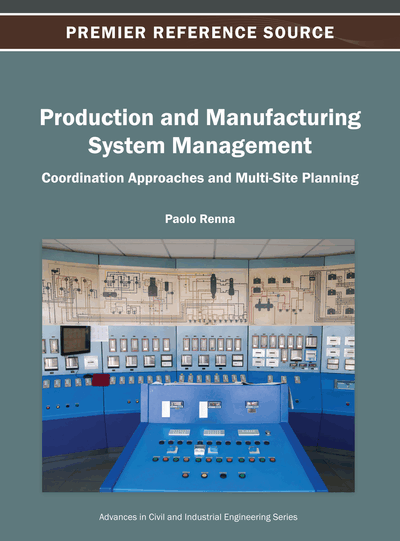# Measures of Risk on Variability with Application in Stochastic Activity Networks

Yousry H. Abdelkader (Alexandria University, Egypt)
DOI: 10.4018/978-1-4666-2098-8.ch012
Available
\$37.50
No Current Special Offers

## Abstract

The author proposes a simple measure of variability of some of the more commonly used distribution functions in the class of New-Better-than-Used in Expectation (NBUE). The measure result in a ranking of the distributions, and the methodology used is applicable to other distributions in NBUE class beside the one treated here. An application to stochastic activity networks is given to illustrate the idea and the applicability of the proposed measure.
Chapter Preview
Top

## Introduction

An important issue that looms high in the management of real life project is that of risk under conditions of uncertainty. “Risk” is may be used as a synonymous with “Variability”, which is best estimated by the variance of the completion time of 'milestone events', including the project completion time, or the project cost, or the resources consumed in its execution or might be R&D see, e.g., (Elamaghraby, 2005; Wang and Hwang, 2007; Durbach and Stewart, 2009). On the other hands, Liu and Liu (2002) defined the expected value and variance for measuring the portfolio return and the risk, respectively. Therefore, one of the most basic measure of risk is the standard deviation or variance. However, other measures of risk may be considered as well see, e.g., (Yitzhaki, 1982; Shailt and Yitzhaki, 1989; Konno and Yamazaki, 1991; Kijima and Ohnishi, 1993; Duffie and Pan, 1997; Levy, 1998; Ringuest et al., 2000; Leshno & Levy, 2002; Tang, 2006; Neiger et al., 2009). Graves and Ringuest (2009) discussed the well known six decision making methods: 1. Mean-Variance, 2. Mean-Semivariance, 3. Mean-Critical Probability, 4. Stochastic Dominance, 5. Almost Stochastic Dominance, and 6. Mean-Gini. They showed the strength and limitations of each technique, and the relationships between the various methodologies. On the other hand, one would prefer a portfolio return with larger degree of asymmetry when the mean and variance are same. A concept of skewness is introduced by Li et al. (2010) to measure the asymmetry of fuzzy portfolio return. The squared coefficient of variationis used to characterize the variability of a distribution see, e.g., (Nuyens & Wierman, 2008). Distributions withare said to have high variability and distributions withare said to have low variability.

Most of the above literature discussed and compared measures of risk in portfolio decision making. In this paper, we propose a measure that assists in comparing variances, hence variability. This measure of risk is used to rank many proposed distribution functions and applying it in project management. Besides, it can be applied in portfolio decision making when different distributions are used. The concept of skewness as well as the squared coefficient of variation are also considered. An application to stochastic activity networks (SANs) is given to illustrate the effectiveness of the proposed measure.

Many probability distributions are discussed in literature as representative of a project activity times. To the best of our knowledge, all suggested distributions fall in the class of 'New Better than Used in Expectation (NBUE)' distributions. For the sake of self-containment we state the distributions that are most frequently discussed in treatises on stochastic activity networks, their mean (denoted byor), variance (denoted byorand skewness (denoted bywhereis the third central moment andis the standard deviation).

## Complete Chapter List

Search this Book:
Reset